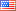# I write the following piece of code

I write the following piece of code:

x = var('x') assume(x,'real') y = var('y') assume(y,'real') z = var('z') assume(z,'real')

expr = abs(x-y)+abs(y-z) >= abs(x-z)

The I run it: sage: bool(expr) False

The expression is obviously mathematically correct. How come Sage returns "False"?2 NoneJohn Palmieri5923 ●18 ●54 ●130 http://www.math.washin...

# I write the following piece of code

I write the following piece of code:

x = var('x')
assume(x,'real')
y = var('y')
assume(y,'real')
z = var('z')
assume(z,'real')assume(z,'real')
expr = abs(x-y)+abs(y-z) >= abs(x-z)abs(x-z)


The I run it:

sage: bool(expr)
FalseFalse


The expression is obviously mathematically correct. How come Sage returns "False"?3 None

# I write wrote the following piece of code

I write the following piece of code:

x = var('x')
assume(x,'real')
y = var('y')
assume(y,'real')
z = var('z')
assume(z,'real')

expr = abs(x-y)+abs(y-z) >= abs(x-z)


The I run it:

sage: bool(expr)
False


The expression is obviously mathematically correct. How come Sage returns "False"?4 None

### Evaluation of triangle inequality

I wrote the following code:

x = var('x')
assume(x,'real')
y = var('y')
assume(y,'real')
z = var('z')
assume(z,'real')

expr = abs(x-y)+abs(y-z) >= abs(x-z)


The Then I run it:

sage: bool(expr)
False


The expression is obviously mathematically correct. How come Sage returns "False"?